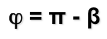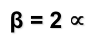# Metric geometry : Angles on the circumference : Central and inscribed

The linear measure abuts the Pythagorean theorem and relationship among these measures on Thales theorem.
The angular measurement from the relations expressed in a circle and with the above can describe the magnitude of geometric figures.
• Central Angle -. It is that which has its apex at the center on the circumference and is understood as the arc.
• Inscribed angle -. is one that has its vertex on the circle and its sides are chords.

An inscribed angle is half of the central angle covered by the same arc.

The sum of angles of a triangle is equal to two right, so the triangle CBP, that is isosceles (two equal angles) relationship will be fulfilled:So we have thatAnd consequently deduce that the central angle is twice the enrolleeIt is easy to generalize this concept to positions of point P that are not so private, and we can decompose the angle in two and apply the same reasoning.

For example, if the point P move along the circumference, the central angle is the sum of the two central angles that can decompose, thus being indifferent point position P.

Metric geometry